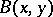# Double negation, law of

(diff) ← Older revision | Latest revision (diff) | Newer revision → (diff)
A logical principle according to which "if it is untrue that A is untrue, A is true" . The law is also called the cancellation law of double negation. In a formalized logical language, the law is expressed asand usually appears in this form (or in the form of the corresponding axiom scheme) in the list of the logical axioms of a given formal theory. In traditional mathematics the law of double negation serves as the logical basis for the performance of so-called indirect proofs in consistent theories according to the following procedure: The assumption that the statementof a given mathematical theory is untrue leads to a contradiction in the theory; since the theory is consistent, this proves that "not A" is untrue, i.e. in accordance with the law of double negation,is true. As a rule, the law of double negation is inapplicable in constructive considerations, which involve the requirement of algorithmic effectiveness of the foundations of mathematical statements. A typical example of such a case is any indirect proof of a statementof the form "for any x there exists a y such that Bx, y is true" ; the last step, viz. the application of the law of double negation, cannot be carried out, since the constructive validity of the statement must entail the existence of an algorithm which, for any, yields a construction ofsuch thatis true. However, an argument which involves the law of double negation does not lead to the construction of any algorithm, and no such algorithm may exist (see also Constructive selection principle).